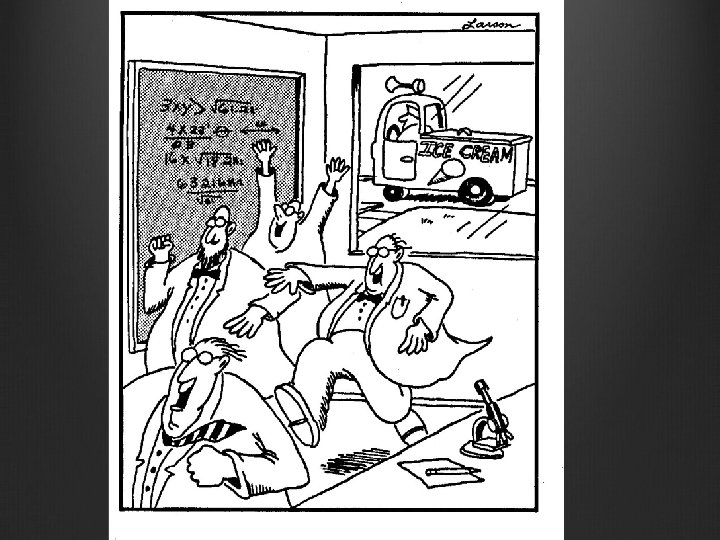quantum information and convex optimization Aram Harrow MIT

• Slides: 25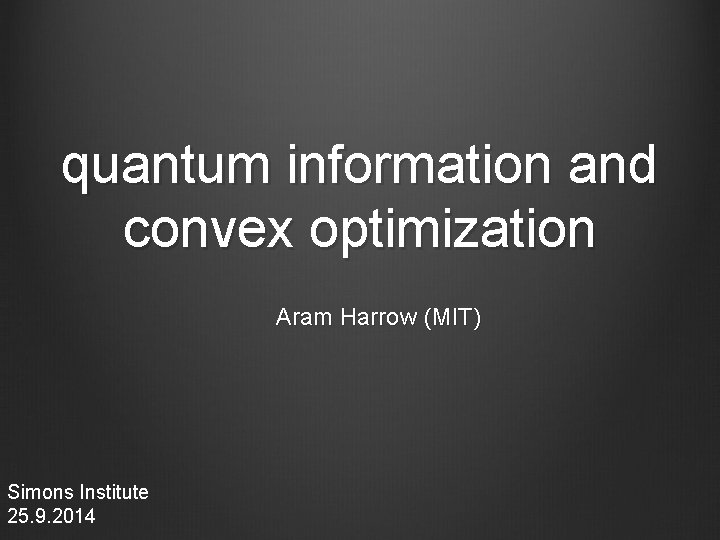quantum information and convex optimization Aram Harrow (MIT) Simons Institute 25. 9. 2014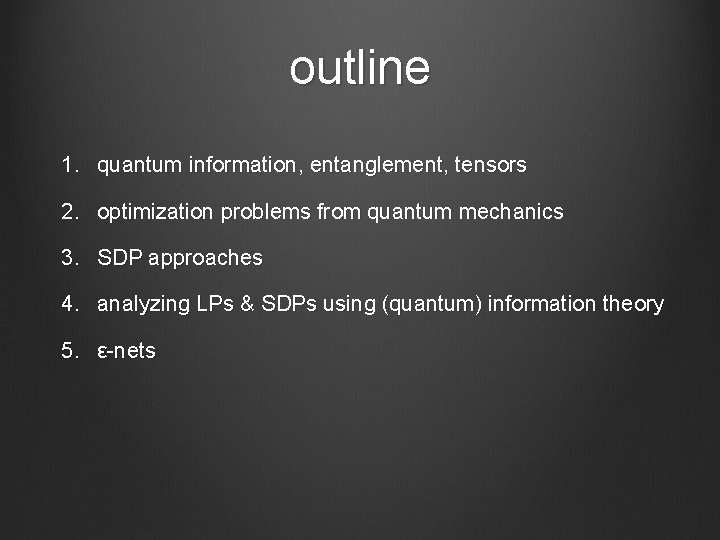outline 1. quantum information, entanglement, tensors 2. optimization problems from quantum mechanics 3. SDP approaches 4. analyzing LPs & SDPs using (quantum) information theory 5. ε-nets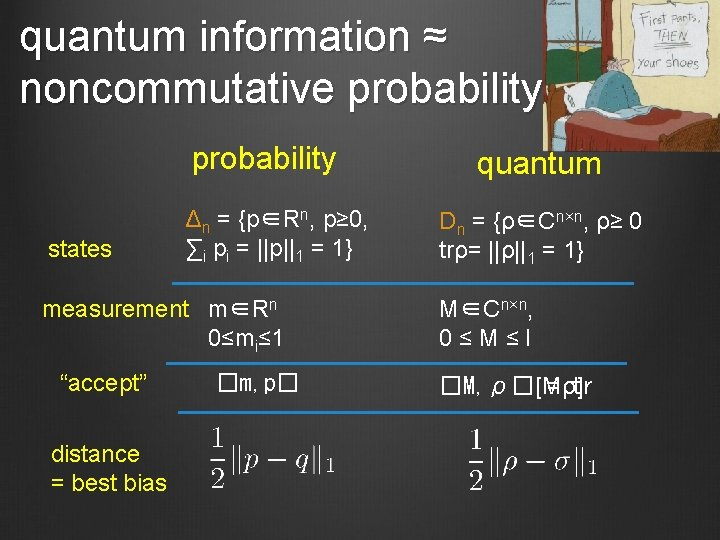quantum information ≈ noncommutative probability states Δn = {p∈Rn, p≥ 0, ∑i pi = ||p||1 = 1} measurement m∈Rn 0≤mi≤ 1 “accept” distance = best bias �m, p� quantum Dn = {ρ∈Cn×n, ρ≥ 0 trρ= ||ρ||1 = 1} M∈Cn×n, 0≤M≤I �M, ρ�[Mρ] = tr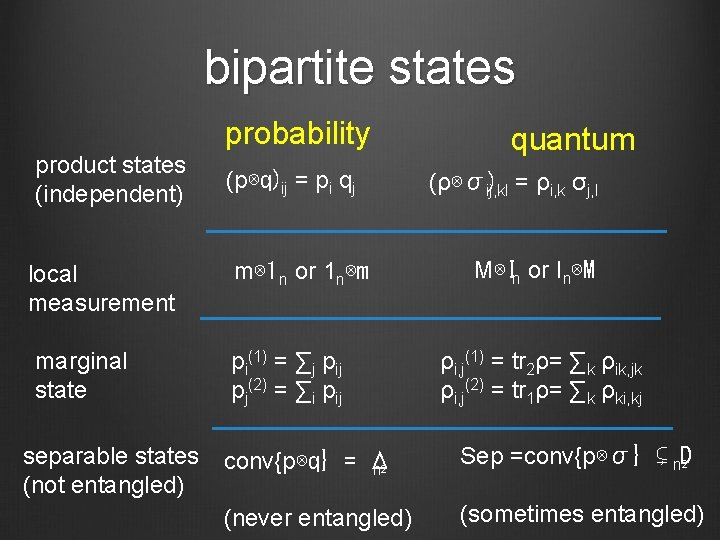bipartite states probability product states (independent) local measurement marginal state separable states (not entangled) (p⊗q)ij = pi qj m⊗ 1 n or 1 n⊗m pi(1) = ∑j pij pj(2) = ∑i pij quantum (ρ⊗σ) ij, kl = ρi, k σj, l M⊗In or In⊗M ρi, j(1) = tr 2ρ= ∑k ρik, jk ρi, j(2) = tr 1ρ= ∑k ρki, kj conv{p⊗q} = Δ n 2 Sep =conv{p⊗σ} ⊊ n. D 2 (never entangled) (sometimes entangled)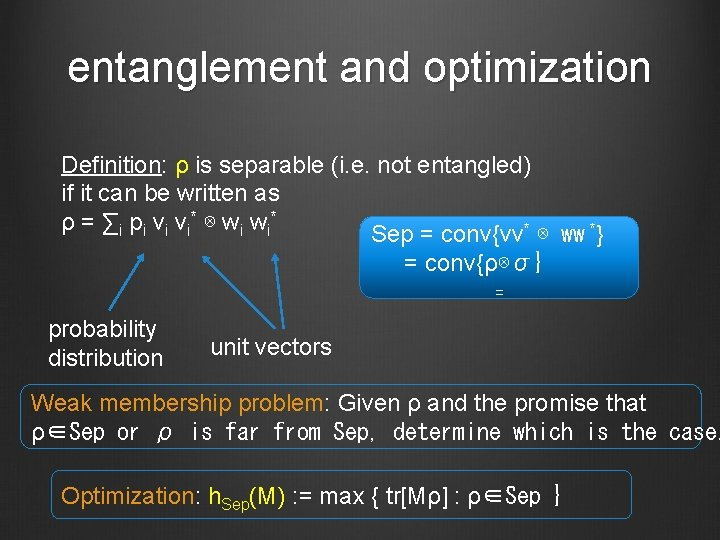entanglement and optimization Definition: ρ is separable (i. e. not entangled) if it can be written as ρ = ∑ i p i v i* ⊗ w i* Sep = conv{vv* ⊗ ww *} = conv{ρ⊗σ} = probability distribution unit vectors Weak membership problem: Given ρ and the promise that ρ∈Sep or ρ is far from Sep, determine which is the case. Optimization: h. Sep(M) : = max { tr[Mρ] : ρ∈Sep }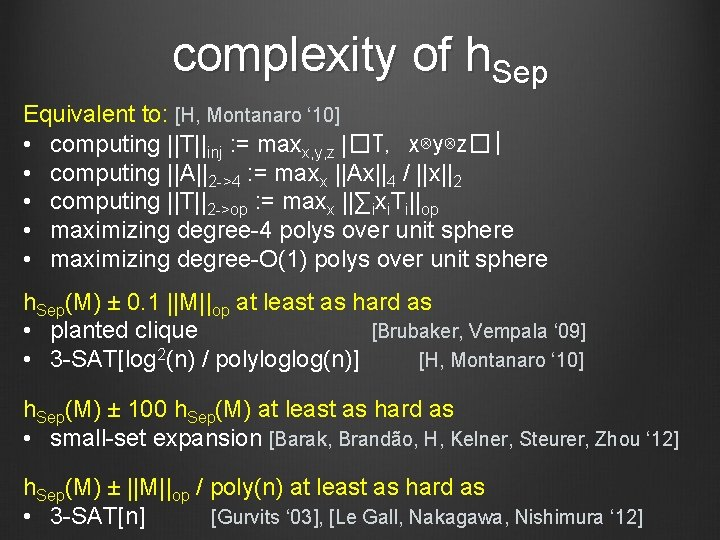complexity of h. Sep Equivalent to: [H, Montanaro ‘ 10] • computing ||T||inj : = maxx, y, z |�T, x⊗y⊗z�| • computing ||A||2 ->4 : = maxx ||Ax||4 / ||x||2 • computing ||T||2 ->op : = maxx ||∑ixi. Ti||op • maximizing degree-4 polys over unit sphere • maximizing degree-O(1) polys over unit sphere h. Sep(M) ± 0. 1 ||M||op at least as hard as • planted clique [Brubaker, Vempala ‘ 09] • 3 -SAT[log 2(n) / polyloglog(n)] [H, Montanaro ‘ 10] h. Sep(M) ± 100 h. Sep(M) at least as hard as • small-set expansion [Barak, Brandão, H, Kelner, Steurer, Zhou ‘ 12] h. Sep(M) ± ||M||op / poly(n) at least as hard as • 3 -SAT[n] [Gurvits ‘ 03], [Le Gall, Nakagawa, Nishimura ‘ 12]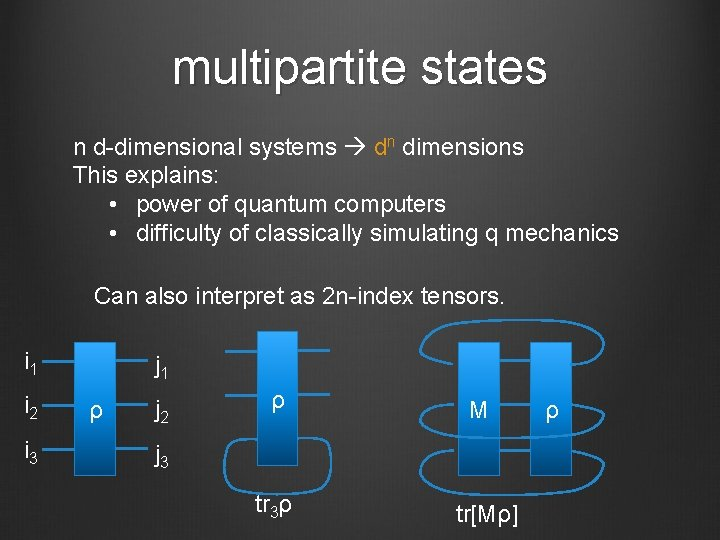multipartite states n d-dimensional systems dn dimensions This explains: • power of quantum computers • difficulty of classically simulating q mechanics Can also interpret as 2 n-index tensors. i 1 i 2 i 3 j 1 ρ j 2 ρ M j 3 tr 3ρ tr[Mρ] ρlocal Hamiltonians Definition: k-local operators are linear combinations of {A 1 ⊗ A 2 ⊗. . . ⊗n : A at most k positions have Ai ≠ I. } intuition: Diagonal case = k-CSPs = degree-k polys Local Hamiltonian problem: Given k-local H, find λmin(H) = minρ tr[Hρ]. QMA-complete to estimate to accuracy ||H|| / poly(n). q. PCP conjecture: . . . or with error ε||H|| QMA vs NP: do low-energy states have good classical descriptions?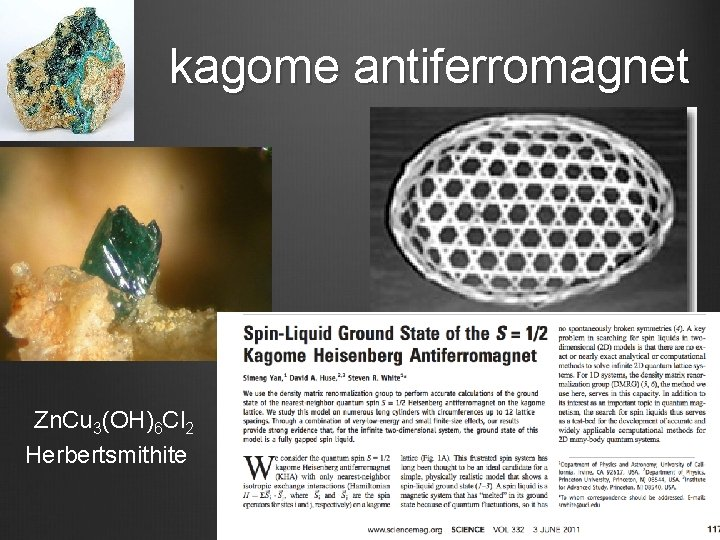kagome antiferromagnet Zn. Cu 3(OH)6 Cl 2 Herbertsmithite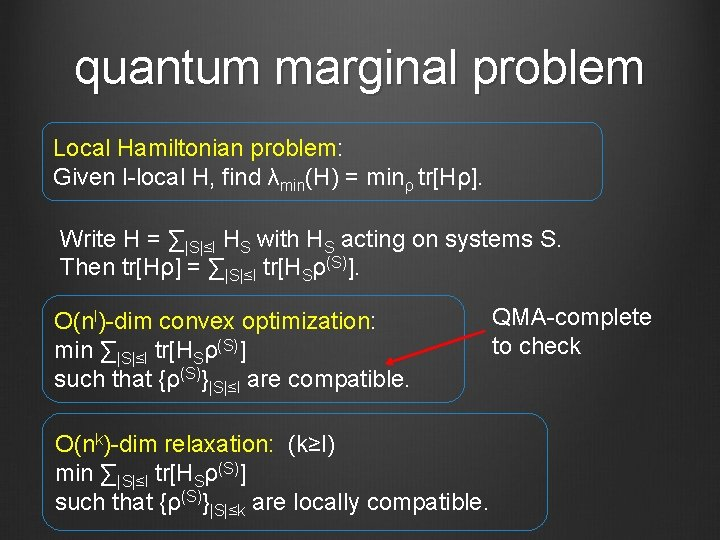quantum marginal problem Local Hamiltonian problem: Given l-local H, find λmin(H) = minρ tr[Hρ]. Write H = ∑|S|≤l HS with HS acting on systems S. Then tr[Hρ] = ∑|S|≤l tr[HSρ(S)]. O(nl)-dim convex optimization: min ∑|S|≤l tr[HSρ(S)] such that {ρ(S)}|S|≤l are compatible. O(nk)-dim relaxation: (k≥l) min ∑|S|≤l tr[HSρ(S)] such that {ρ(S)}|S|≤k are locally compatible. QMA-complete to checkOther Hamiltonian problems Properties of ground state: i. e. estimate tr[Aρ] for ρ = argmin tr[Hρ] reduces to estimating λmin(H + μA) Non-zero temperature: Estimate log tr e-H and derivatives #P-complete, but some special cases are easier (Noiseless) time evolution: Estimate matrix elements of ei. H BQP-complete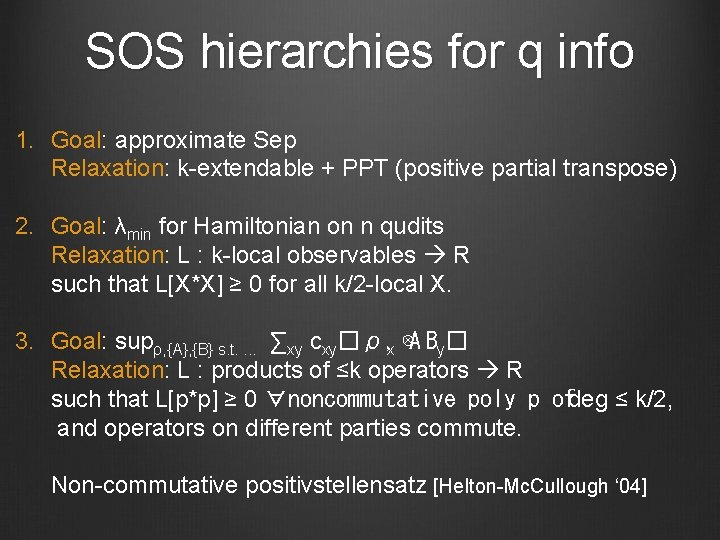SOS hierarchies for q info 1. Goal: approximate Sep Relaxation: k-extendable + PPT (positive partial transpose) 2. Goal: λmin for Hamiltonian on n qudits Relaxation: L : k-local observables R such that L[X*X] ≥ 0 for all k/2 -local X. 3. Goal: supρ, {A}, {B} s. t. . ∑xy cxy�ρ, x ⊗A By� Relaxation: L : products of ≤k operators R such that L[p*p] ≥ 0 ∀noncommutative poly p ofdeg ≤ k/2, and operators on different parties commute. Non-commutative positivstellensatz [Helton-Mc. Cullough ‘ 04]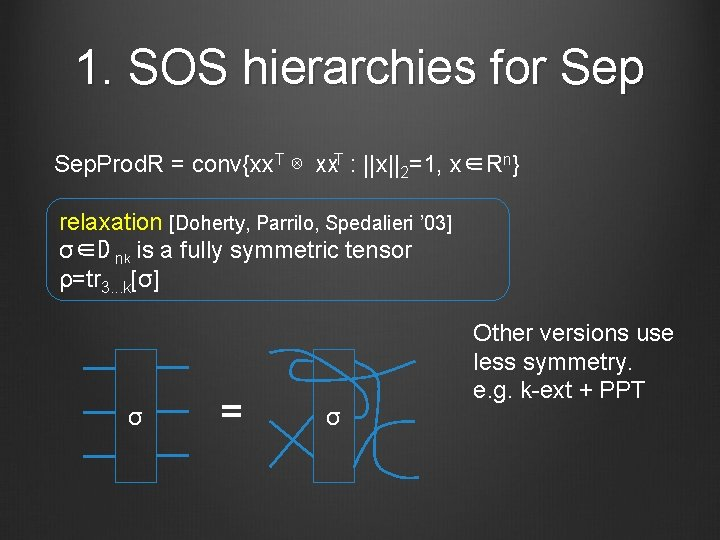1. SOS hierarchies for Sep. Prod. R = conv{xx. T ⊗ xx. T : ||x||2=1, x∈Rn} relaxation [Doherty, Parrilo, Spedalieri ’ 03] σ∈D nk is a fully symmetric tensor ρ=tr 3. . . k[σ] σ = σ Other versions use less symmetry. e. g. k-ext + PPT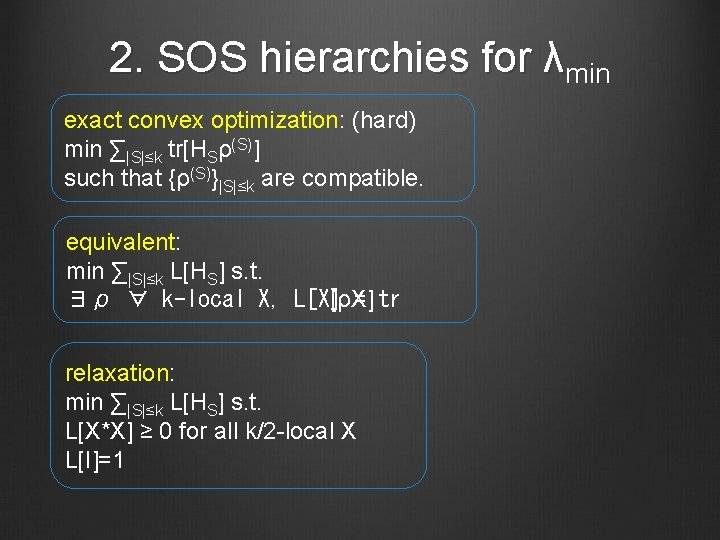2. SOS hierarchies for λmin exact convex optimization: (hard) min ∑|S|≤k tr[HSρ(S)] such that {ρ(S)}|S|≤k are compatible. equivalent: min ∑|S|≤k L[HS] s. t. ∃ρ ∀ k-local X, L[X][ρX] = tr relaxation: min ∑|S|≤k L[HS] s. t. L[X*X] ≥ 0 for all k/2 -local X L[I]=1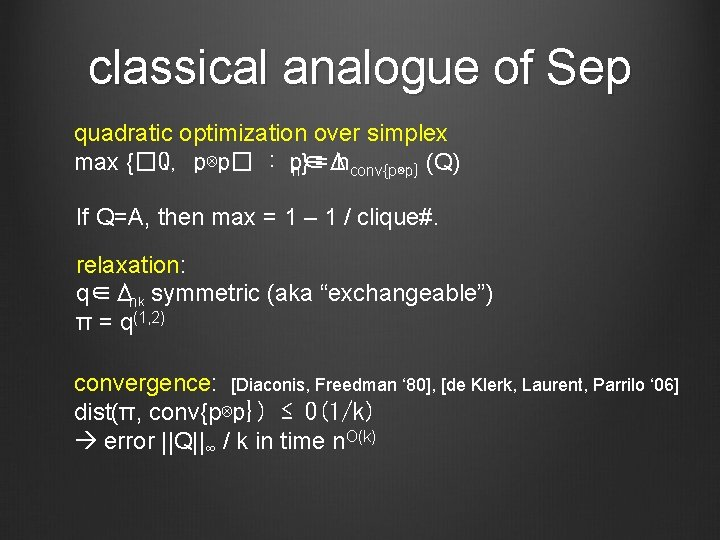classical analogue of Sep quadratic optimization over simplex max {�Q, p⊗p� : p∈Δ n} = hconv{p⊗p} (Q) If Q=A, then max = 1 – 1 / clique#. relaxation: q∈Δnk symmetric (aka “exchangeable”) π = q(1, 2) convergence: [Diaconis, Freedman ‘ 80], [de Klerk, Laurent, Parrilo ‘ 06] dist(π, conv{p⊗p}) ≤ O(1/k) error ||Q||∞ / k in time n. O(k)Nash equilibria Non-cooperative games: B Players choose strategies p. A ∈ Δ m, p ∈ Δ n. A ⊗ p. B� Receive values �V , p and �V , p. A B Nash equilibrium: neither player can improve own value ε-approximate Nash: cannot improve value by > ε Correlated equilibria: Players follow joint strategy p. AB ∈ Δ mn. AB� Receive values �V , p and �V , p. A B Cannot improve value by unilateral change. • Can find in poly(m, n) time with LP. • Nash equilibrium = correlated equilibrum with p = p. A ⊗ p. B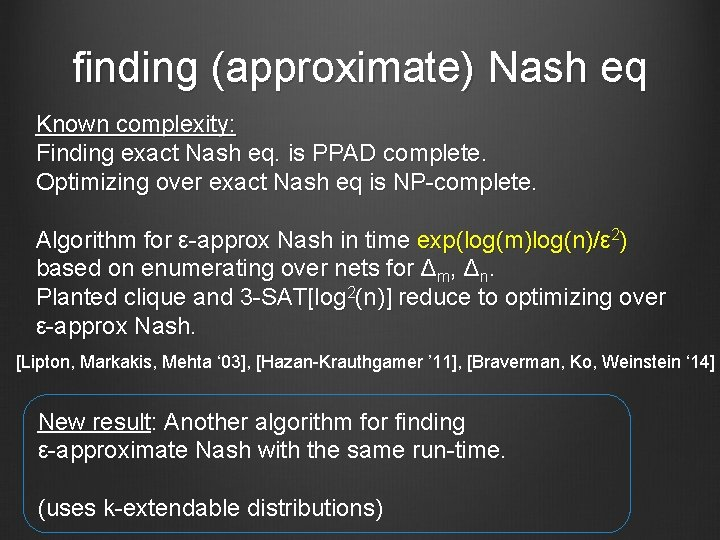finding (approximate) Nash eq Known complexity: Finding exact Nash eq. is PPAD complete. Optimizing over exact Nash eq is NP-complete. Algorithm for ε-approx Nash in time exp(log(m)log(n)/ε 2) based on enumerating over nets for Δm, Δn. Planted clique and 3 -SAT[log 2(n)] reduce to optimizing over ε-approx Nash. [Lipton, Markakis, Mehta ‘ 03], [Hazan-Krauthgamer ’ 11], [Braverman, Ko, Weinstein ‘ 14] New result: Another algorithm for finding ε-approximate Nash with the same run-time. (uses k-extendable distributions)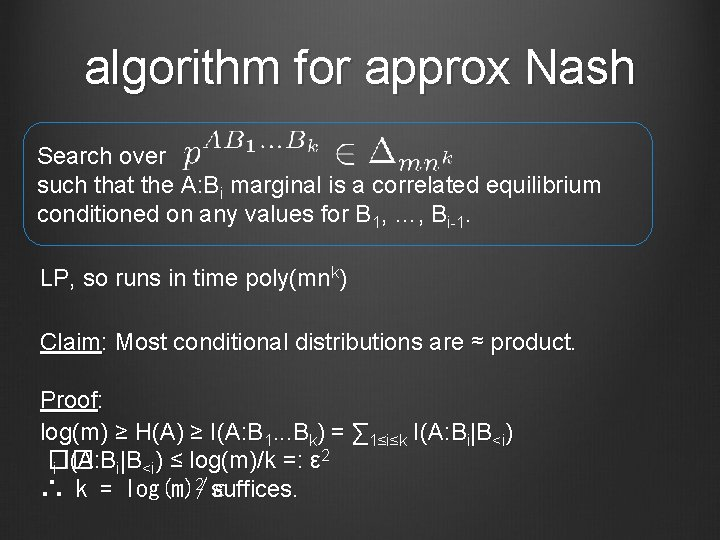algorithm for approx Nash Search over such that the A: Bi marginal is a correlated equilibrium conditioned on any values for B 1, …, Bi-1. LP, so runs in time poly(mnk) Claim: Most conditional distributions are ≈ product. Proof: log(m) ≥ H(A) ≥ I(A: B 1. . . Bk) = ∑ 1≤i≤k I(A: Bi|B<i) 2 �� i I(A: Bi|B<i) ≤ log(m)/k =: ε 2 suffices. ∴ k = log(m)/εSOS results for h. Sep(n, m) = conv{ρ1 ⊗. . . ⊗m ρ : ρm ∈ Dn} Sep. Sym(n, m) = conv{ρ⊗m : ρ ∈ Dn} bipartite doesn’t match hardness Thm: If M =∑i Ai ⊗B i with ∑i |Ai| ≤ I, each |Bi| ≤ I, then h. Sep(n, 2)(M) ≤ hk-ext(M) ≤ h. Sep(n, 2)(M) + c (log(n)/k)1/2 [Brandão, Christandl, Yard ’ 10], [Yang ’ 06], [Brandão, H ’ 12], [Li, Winter ‘ 12] multipartite Thm: ε-approx to h. Sep. Sym(n, m)(M) in time exp(m 2 log 2(n)/ε 2). ε-approx to h. Sep(n, m)(M) in time exp(m 3 log 2(n)/ε 2). [Brandão, H ’ 12], [Li, Smith ’ 14] ≈matches Chen-Drucker hardnessSOS results for λmin H = E(i, j)∈E Hi, j acts on (Cd)⊗n such that • each ||Hi, j|| ≤ 1 • |V| = n • (V, E) is regular • adjacency matrix has ≤r eigenvalues ≥poly(ε/d) Theorem λmin(H) ≈ε h. Sep(d, n)(H) and can compute this to error ε with r∙poly(d/ε) rounds of SOS, i. e. time nr∙poly(d/ε). [Brandão-H, ’ 13] based on [Barak, Raghavendra, Steurer ‘ 11]net-based algorithms M =∑i∈[m] Ai ⊗B i with ∑i Ai ≤ I, each |Bi| ≤ I, Ai ≥ 0 hierarchies estimate h. Sep(M) ±ε in time exp(log 2(n)/ε 2) h. Sep(M) = maxα, βtr[M(α⊗β)] max = p∈S ||p||B S = {p : ∃α s. t. i =ptr[Aiα]} ⊆Δm ||x||B = ||∑i xi Bi||op Lemma: ∀p∈Δm ∃q k-sparse (each i q= integer / k) ||p-q||B ≤ c(log(n)/k)1/2. Pf: matrix Chernoff [Ahlswede-Winter] Algorithm: Enumerate over k-sparse q • check whether ∃p∈S, ||p-q|| B ≤ε • if so, compute ||q||B Performance 2, m=poly(n) k ≃ log(n)/ε run-time O(mk) = exp(log 2(n)/ε 2)nets for Banach spaces X: A->B ||X||A->B = sup ||Xa||B / ||a||A operator norm ||X||A->C->B = min {||Z||A->C ||Y||C->B : X=YZ} factorization norm Let A, B be arbitrary. C = l 1 m Only changes are sparsification (cannot assume m≤poly(n)) and operator Chernoff for B. Type 2 constant: T 2(B) is smallest λ such that result: estimated in time exp(T 2(B)2 log(m)/ε 2)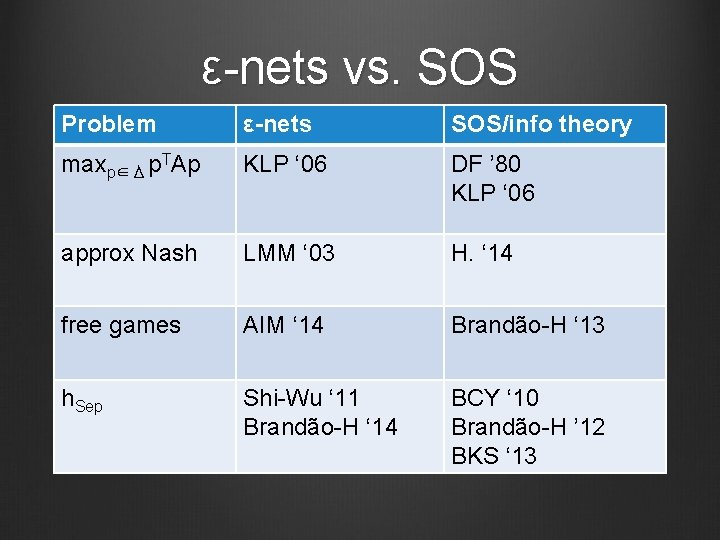ε-nets vs. SOS Problem ε-nets SOS/info theory maxp∈Δ p. TAp KLP ‘ 06 DF ’ 80 KLP ‘ 06 approx Nash LMM ‘ 03 H. ‘ 14 free games AIM ‘ 14 Brandão-H ‘ 13 h. Sep Shi-Wu ‘ 11 Brandão-H ‘ 14 BCY ‘ 10 Brandão-H ’ 12 BKS ‘ 13questions / references Application to 2 ->4 norm and small-set expansion. Matching quasipolynomial algorithms and hardness. simulating noisy/low-entanglement dynamics conditions under which Hamiltonians are easy to simulate Relation between hierarchies and nets Meaning of low quantum conditional mutual information Hardness/connections 1001. 0017 Relation to 2 ->4 norm, SSE 1205. 4484 SOS for h. Sep 1210. 6367 SOS for λmin 1310. 0017